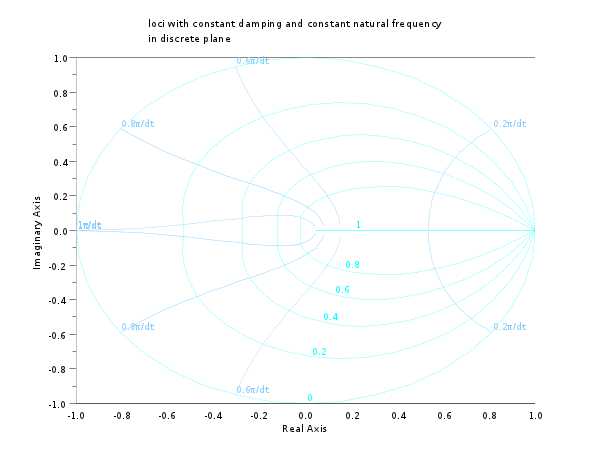Scilab Home page | Wiki | Bug tracker | Forge | Mailing list archives | ATOMS | File exchange
Change language to: Français - Português - 日本語

See the recommended documentation of this function

Scilab help >> CACSD > zgrid

# zgrid

zgrid plot

### Calling Sequence

```zgrid()
zgrid(zeta,wn [,colors])
zgrid(['new',] zeta,wn [,colors])
zgrid(zeta,wn [,'new'] [,colors])```

### Arguments

zeta

array of damping factors. Only values in ```[0 1]``` are taken into account. The default value is `0:0.1:1`.

wn

array of normalized natural frequencies (factors of π/dt). Only values in `[0 1]` are taken into account. The default value is `0:0.1:1`.

colors

a scalar or an 2 element array with integer values (color index).

### Description

plots z-plane grid lines: lines of constant damping factor (selection given by `zeta`) and natural frequency (selection given by `wn`) are drawn in within the unit Z-plane circle.

Iso-frequency curves are shown in on the interval [0,%pi/dt].

The `colors` argument may be used to assign a color for constant damping ratio curves (`colors(2)`) and for frequency curves (`colors(1)`).

The `zgrid` function is often used to draw a grid for evens root locus of continuous time linear systems. In such a case the `zgrid` function should be called after the call to evans. For continuous time linear systems one should use sgrid function instead.

The optional argument `'new'` can be used to erase the graphic window before plotting the grid.

### Examples

```//zgrid
clf();zgrid(0:0.2:1,[0.2 0.6 0.8 1])``````//zgrid with discrete time system  root locus
z=poly(0,'z')
H=syslin(0.01,(0.54-1.8*z+2.9*z^2-2.6*z^3+z^4)/(0.8+0.78*z-0.1*z^2+0.9*z^3+z^4))
clf();evans(H,1000);zgrid(0:0.1:0.5)```• evans — Evans root locus
• sgrid — s-plane grid lines.
• freson — peak frequencies
• datatips — Tool for placing and editing tips along the plotted curves.